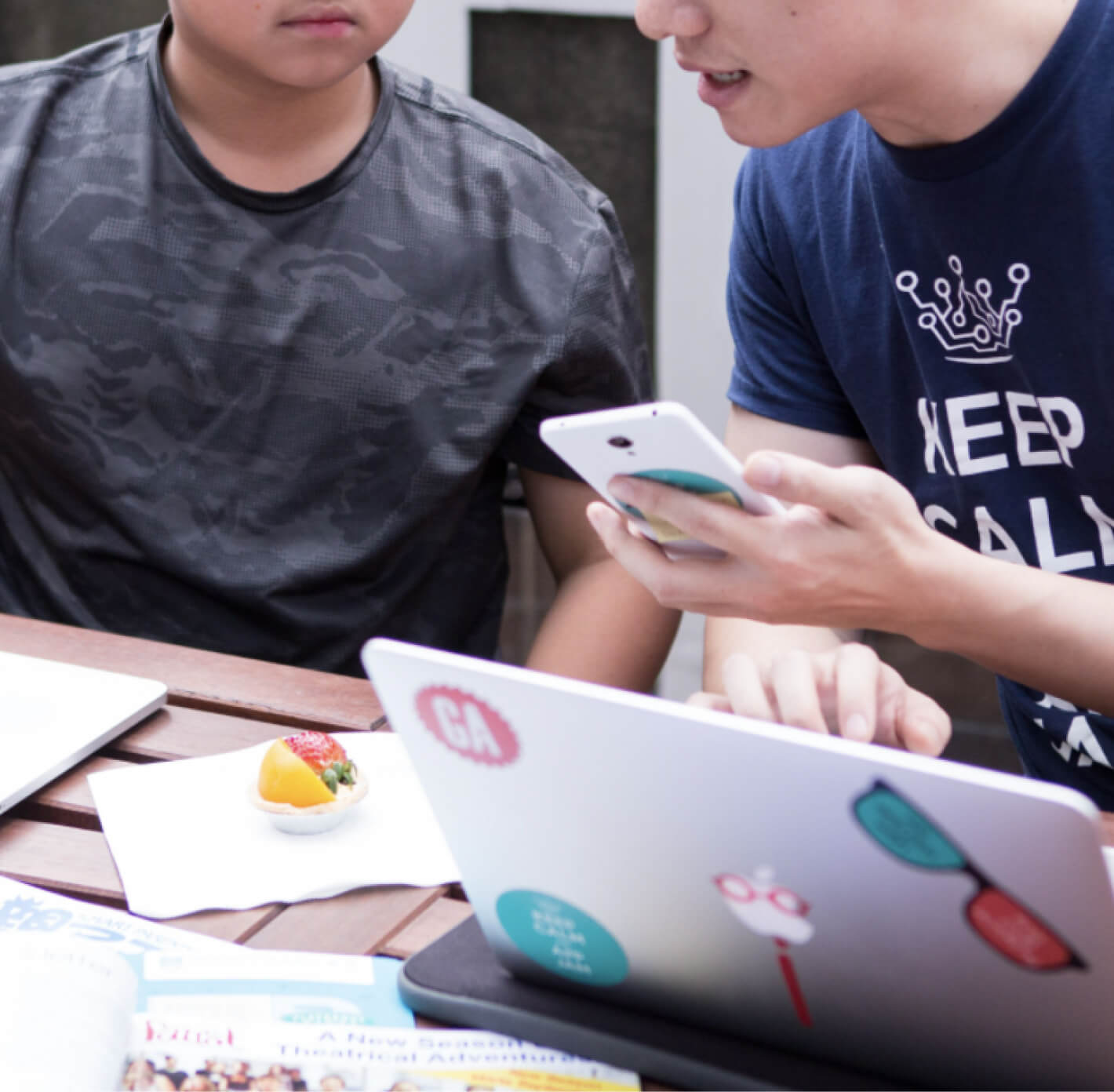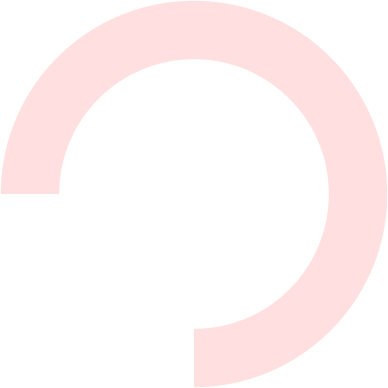Python 1 - Programming with Python: Introduction (12yo+)

Designed for any student curious about the power of computers and technology, the Python 101 course introduces the basics of Python and computer science by programming a series of fun games and rewarding code challenges. In addition to Python syntax and computer science concepts, students also learn to think about problems logically and solve them systematically. The course also introduces Python libraries to students, laying a solid foundation towards many more powerful applications of Python at higher levels.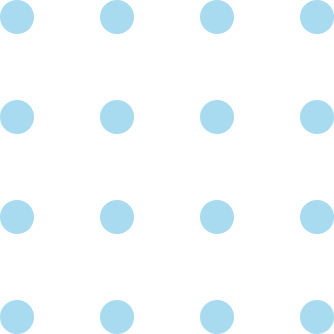Prerequisite

None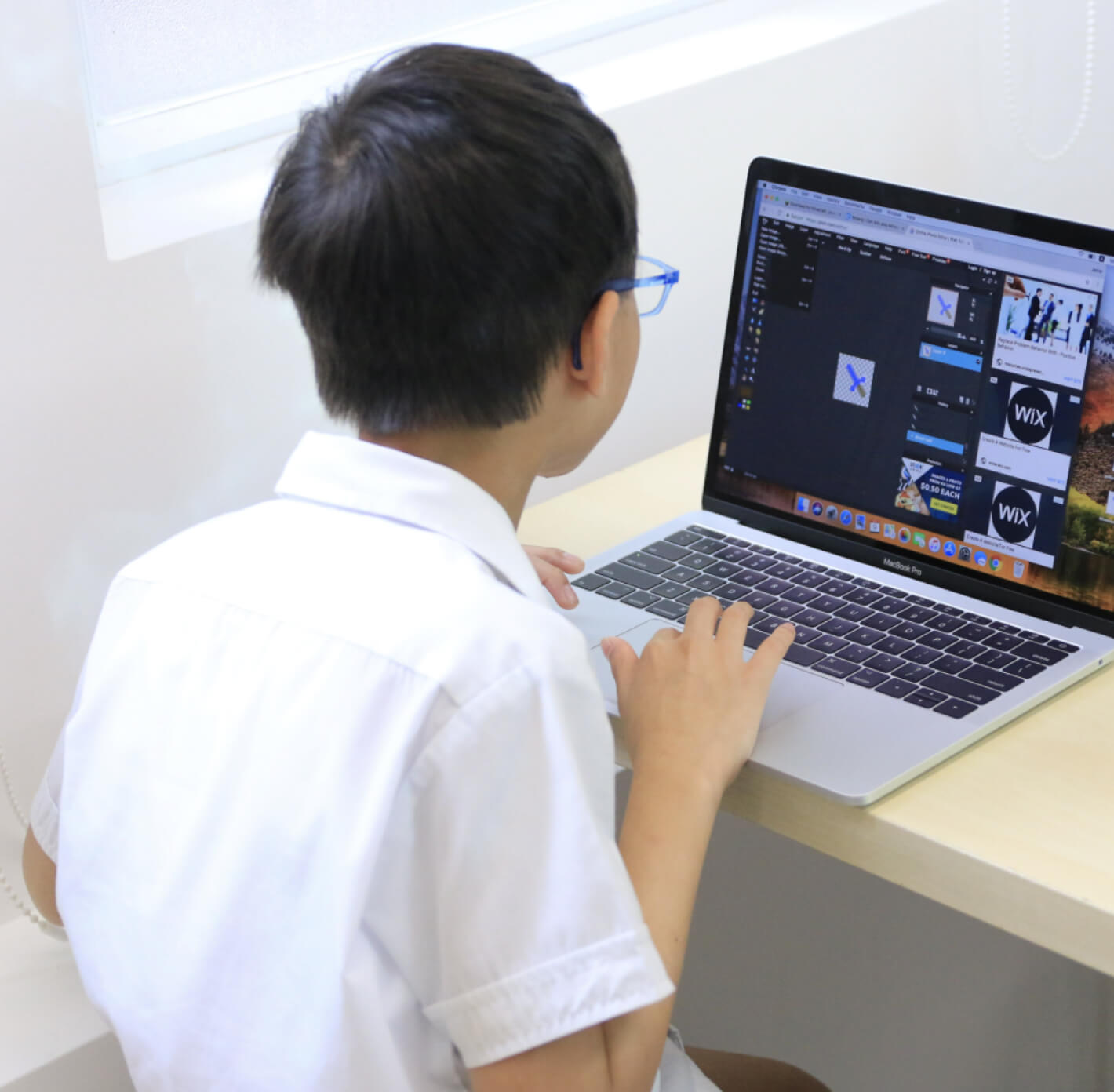Course Structure

Lecture, Project-based learning, instructor-led brainstorming sessions

Introduction to the Python Language

• Introduction to command line basics
• Introduction to the Python syntax and data types
• Hands-on: creating simple interactive projects with Python

Simple Python Programming

• Introduction to loops, functions and control flow in Python
• Familiarizing with the Python Turtle library
• Hands-on: creating simple games and animations with Python and Python turtle

Data Structures in Python

• Introduction to data structures and manipulating files in Python
• Familiarizing with control flow design and code planning
• Exercises and programming challenges to solidify Python knowledge
• Hands-on: creating games and functional programs with Python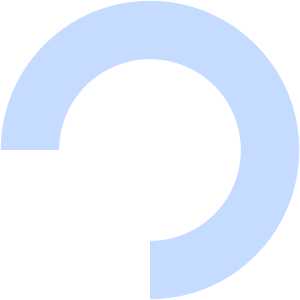Schedule

\$2,240